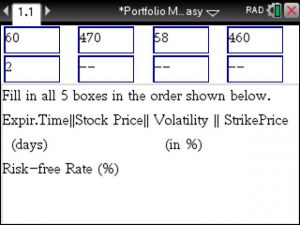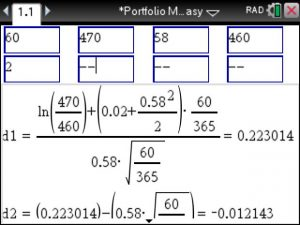## Tinspire CX : Black Scholes Pricing – Step by Step

The Black Scholes Option Pricing may be used to compute the fair market value of options, its computation requires some level of mathematical analysis. If you own a Tinspire CX you can easily compute the Black Scholes Put and Call pricing – step by step – using the Portfolio Made Easy app at https://www.tinspireapps.com/?a=PIME . Just follow the steps below.

Select 1: Black Scholes : Call & Put PriceEnter the expiration date (in days), the stock price , its volatility (in %) , the strike price and the risk-free rate (in%)Notice how the value are plugged into the Black Scholes formula. Notice how the 60 days are automatically divided by 365 days to turn the 60 days into a fraction. Additionally, the volatility and the risk-free rate re expressed as decimals.After computing the parameters d1, d2 and the values of the normal distribution the Call price C is determined, here C = 49.4468Lastly , the Put Price is computed, here P=37.937The computation is easily accomplished simply by entering the given values, and it is always correct 😉

Posted on Categories finances, option pricing